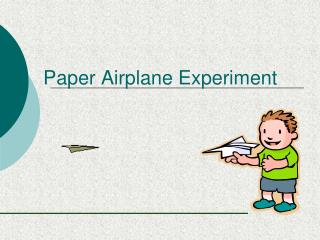DownloadDownload PresentationPaper Airplane Experiment

# Paper Airplane Experiment

Download Presentation## Paper Airplane Experiment

- - - - - - - - - - - - - - - - - - - - - - - - - - - E N D - - - - - - - - - - - - - - - - - - - - - - - - - - -
##### Presentation Transcript

1. Paper Airplane Experiment

2. Research • Have you flown a paper airplane before? (Hopefully not in this class) • Do you always use the same type of paper? • Do you always use the same design? • Do you want it to fly straight or do tricks?

3. Introduction: • We are going to design an experiment to test paper airplane flight distance. • We want the planes to fly as far as they can. • We need to think about how we are going to design and perform the experiment. • What things do we need to think about? (Think about the steps of the Scientific Method)

4. Problem: • What question are we trying to answer? • We want to design an experiment to test how the addition of paper clips will affect the flight distance of the paper airplane. • How does adding paper clips to a paper airplane affect its flight?

5. Hypothesis: • What do you think is going to happen? • How do you think the addition of paper clips to the paper airplane affect its flight distance? • Does the placement of the clips matter? • If paper clips are added to the (location)_ of the paper airplane, then ___________ _________________________________.

6. Materials: • What do we need to perform this experiment? • Make a list of materials: • Paper • Measuring tape • Meter Stick • Paper clips • Pen/Pencil

7. Procedure: • How are we going to perform the experiment? • What do we need to do? • What needs to be kept constant? • What is our control? • What is our independent variable going to be? • Where are we going to perform the experiment? • What are we going to observe? How? • This should be written as a list of numbered steps.

8. Procedure • Get paper clips, lab notebook and a meter stick. • Make the paper airplane. • Gather materials and head out to your assigned runway. • Fly your airplane, measure flight distance, and record your data in your notebook.

9. Procedure • Throw plane from behind the starting line each time. Launch at same angle and with same speed each time. • Add a paper clip for each new launch. Record data • Clean up and return to classroom

10. Observations: • Data Table with measurements from the experiment. • Include headings and labels.

11. Lab Report Write Up • Must include: • Title page: Title, name, class, date • Introduction: one paragraph about why we did this experiment • Problem: What question did we want to answer? • Hypothesis: What you think will happen and why? • Materials: A list of materials used • Procedure: Step by step explanation of what you did to perform the experiment. • Data Table and Graph: Include labels • Conclusion: Explain your results • Questions: Answer the questions about the lab in complete sentences. Click on the lab notebook to see a sample lab report

12. Making a graph of your data • Let’s review independent and dependent variables. • The independent variable is what the scientist changes during an experiment. • The dependent variable is what happens due to what is changed. • Remember: The growth of the flower is dependent upon the amount of water it receives.

13. 10 8 6 Flight Distance (meters) 4 2 0 0 1 2 3 4 5 6 Number of paper clips Making the graph How adding paper clips affects paper airplane flight distance. 1. Create a title. 3. Determine interval and label for y-axis. 4. Plot your data. 5. Connect the data points. 2. Determine interval and label for x-axis.

14. Conclusion: • What did you learn during the experiment? • Does your data make sense? • Was your hypothesis correct? Explain why or why not? • Did you do anything wrong? • Is there anything that you would have or should have done differently? • Your conclusion should be 3 to 4 paragraphs long.

15. Questions: • What is a control? What was the control in this experiment? • What is a constant? What were the constants in this experiment? • What is an independent variable? What was the independent variable in this experiment? • What is a dependent variable? What was the dependent variable in this experiment? • Should your results be the same as others? Why or why not? The answers to the questions must be in complete sentences!